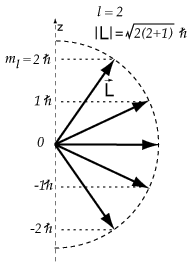# An electron is in the hydrogen atom with n = 3. |L| = sqrt6 ℏ . Which is a possible angle between vecL and the z axis?

May 26, 2017

The only angle that satisfies the criteria is 65.9°.

#### Explanation:

In the Schrödinger equation for the hydrogen atom, the squared orbital angular momentum operator, ${\hat{L}}^{2}$, corresponds to the eigenvalue, l(l+1)ℏ^2, according to this formula:

bar(ul(|color(white)(a/a) ul(hat(L)^2)Y_(l)^(m_l)(theta,phi) = ul(l(l+1)ℏ^2)Y_(l)^(m_l)(theta,phi) color(white)(a/a)|))" "

or

color(blue)(bar(ul(|color(white)(a/a) ul(hat(L)) harr ul(sqrt(l(l+1))ℏ) color(white)(a/a)|)))" "

where

• $l$ is the angular momentum quantum number.
• ${Y}_{l}^{{m}_{l}} \left(\theta , \phi\right)$ is the spherical harmonic wave function for the orbital of a given $l$ and ${m}_{l}$.
• ${m}_{l}$ is the magnetic quantum number, and the projection of $l$ along the $z$ axis.

The $z$-component of the angular momentum similarly corresponds to the eigenvalue m_lℏ according to:

bar(ul(|color(white)(a/a)ul(hatL_z)Y_(l)^(m_l)(theta,phi) = ul(m_lℏ)Y_(l)^(m_l)(theta,phi)color(white)(a/a)|))" "

or

color(blue)(bar(ul(|color(white)(a/a)ul(hatL_z) harr ul(m_lℏ) harr |L|cosθcolor(white)(a/a)|)))" "

where

• ${m}_{l}$ is the magnetic quantum number and
• θ is the angle that $\vec{L}$ makes with the $z$-axis, or the angle of $\hat{L}$ with respect to ${m}_{l}$.Thus

θ = cos^"-1"(m_l/|L|)

Some possibilities for $n = 3$ are

bb(lcolor(white)(mmm)|L|//ℏ)
$0 \textcolor{w h i t e}{m l} \sqrt{0 \left(1\right)} = 0$
$1 \textcolor{w h i t e}{m l} \sqrt{1 \left(2\right)} = \sqrt{2}$
$2 \textcolor{w h i t e}{m l} \sqrt{2 \left(3\right)} = \sqrt{6}$

It appears that our electron is a $\text{d}$ electron ($l = 2$).

and

|L| = sqrt6ℏ = 2.4495ℏ

The possible angles for a $\text{d}$ electron are

bb(color(white)(m)|L|"/"ℏcolor(white)(mm)m_l//ℏcolor(white)(ml)cosθcolor(white)(mmm)θ)

$2.4495 \textcolor{w h i t e}{m m l} 2 \textcolor{w h i t e}{m m l} 0.8165 \textcolor{w h i t e}{m l l} 35.26$
$2.4495 \textcolor{w h i t e}{m m l} 1 \textcolor{w h i t e}{m m l} 0.4082 \textcolor{w h i t e}{m l l} 65.90$
$2.4495 \textcolor{w h i t e}{m m l} 0 \textcolor{w h i t e}{m m l} 0 \textcolor{w h i t e}{m m m m l} 90$
$2.4495 \textcolor{w h i t e}{m m} \text{-1"color(white)(mm)"-0.4082} \textcolor{w h i t e}{m} 114.09$
$2.4495 \textcolor{w h i t e}{m m} \text{-2"color(white)(mm)"-0.8165} \textcolor{w h i t e}{m} 144.74$

The only angle that agrees with those given in the question is 65.90°.